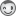## (sort of) weighted average

Hello,

Can anyone tell me how to calculate weighted averages in this example:

```data premium;
datalines;
1 1 1000
2 0.5 500run;
```

Customers 1 and 2 both payed the same premium, but no. 2 was insured for only six months.
They the same annual premium, so in my opinion the average premium per person is 1000.
However, using weight does not work correctly in this case:

```proc means mean;
weight fraction;
run;
```

It returns 833 as the average premium.
Currently I solve this by exporting means output, summing fraction and premium and divide.

Does anyone know of an easy, better way - without calculating new variables in the datastep (there are many variables)?

Thanks,
Eric

1 ACCEPTED SOLUTION

Accepted Solutions

## Re: (sort of) weighted average

Chris was faster and is correct.

What you want is the weighted mean of the annualized premiums with the fractions being the weights. This, indeed, amounts to (sum of premiums)/(sum of fractions) and hence can be calculated as shown by Chris or similarly as follows:

``````proc sql;
quit;``````
19 REPLIES 19

## Re: (sort of) weighted average

You could use PROC SQL:

``````proc sql;
quit;
``````

## Re: (sort of) weighted average

Thanks for asking your question on the communities here.  I see you've also asked it in other places (SAS-L, blog comments) so I want to add some more of that context.

It seems like you really want an "annualized premium" -- what each customer is paying per annum (year) as a rate.  If the term is something different that 1.0 years, you need a formula to calculate what the annual rate is.

I've seen this answer on SAS-L by "retired mainframer":

``````data null;
do until (eof);
count+1;
end;
average = sum/count;
put average=;
run;
``````

The SQL approach by @FreelanceReinh might also serve your purpose.

## Re: (sort of) weighted average

Thanks Chris and Reinhard,

The next step is that I want the premiumpaid by No 2 to weigh less than the premium paid by No 1.

If I change the case to

The average annual premium should be (900 + 600) / 1.5 = 1000.

In Reinhard's case it is (900 + 1200) / 2 = 1050.

I can see it can be solved using Chris' script. But then I have dozens of variables I want to treat this way...

But thanks!! for your quick replies.

Cheers,

Eric

## Re: (sort of) weighted average

If you prefer SQL, I think this accomplishes something similar:

``````PROC SQL;
CREATE TABLE WORK.Avgs AS
SELECT   (COUNT(t1.id)) AS N,
(SUM(t1.fraction)) AS AnnumTotal,
/* AnnualizedAvg */
calculated PremiumTotal / calculated AnnumTotal AS AnnualizedAvg
QUIT;
``````

thx!

## Re: (sort of) weighted average

Chris was faster and is correct.

What you want is the weighted mean of the annualized premiums with the fractions being the weights. This, indeed, amounts to (sum of premiums)/(sum of fractions) and hence can be calculated as shown by Chris or similarly as follows:

``````proc sql;
quit;``````

## Re: (sort of) weighted average

Thanks Chris and Reinhard. I feel a macro coming up...

## Re: (sort of) weighted average

No, wait a minute! You don't need a macro. I can prepare an array solution for you.

## Re: (sort of) weighted average

So, you have more variables like PREMIUM, let's say three, for example: PREMIUM, VAR2, VAR3. And let's assume for a moment that the weights ("fractions") are the same for all these variables. (Or do you have individual fractions for each of them?)

In this case you could extend the DOW loop approach cited by Chris. But I'm afraid there is a correction necessary: The SET statement must be moved inside the DO-UNTIL loop (that's the trick of the DOW loop after all), otherwise we have an infinite loop.

Now you can calculate the target variables, let's call them MEAN_PREMIUM, MEAN_VAR2 and MEAN_VAR3, as follows:

``````data premium1;
input id fraction premium var2 var3;
cards;
1 1   1000 900 500
2 0.5 500  600 400
;

%let n=3; /* number of variables like PREMIUM */

data _null_;
array s[&n];
do until (eof);
do i=1 to &n;
s[i]+p[i];
end;
wgt+fraction;
end;
do i=1 to &n;
avg[i] = s[i]/wgt;
end;
put avg(*)=;
run;
``````

If you need individual fractions for each of the three variables, we could introduce a fourth array, which would be no problem at all.

## Re: (sort of) weighted average

Reinhard, Thanks for your extensive reply. It is definitely something to apply in my case!

## Re: (sort of) weighted average

Perhaps I am not understanding the problem, but I have to disagree with several of the submitted "solutions." The solution that you accepted as correct does not seem correct to me. Both @ChrisHemedinger and @FreelanceReinh suggested implementations of the formula sum(premium)/sum(fraction).  That is not the same as mean(premium/fraction), which I think is the correct formula.

## Re: (sort of) weighted average

Hello (again) Rick,

In my data I have premiums and fractions of a year that a customer was insured.

So in my opinion the average premium per customer is sum(premium)/sum(fraction). This is a sort of weighted average, unfortunately not supported by the MEANS procedure.

So being the customer (who is always right), I think the solutions are correct.

Cheers, Eric

## Re: (sort of) weighted average

@Rick_SAS: Well, mean(premium/fraction) was my first suggestion, but then @EH replied "I want the premium paid by No 2 to weigh less than the premium paid by No 1" and presented an example calculation that led us to the sum(premium)/sum(fraction) solution.

## Re: (sort of) weighted average

There is a similar question, marked as solved, but I cannot find the solution.

https://communities.sas.com/t5/SAS-Procedures/Weighted-average/m-p/24378#U24378

In the solution is referred to the VARDEF option, but that does not apply to the weighted mean calculation.

Discussion stats
• 19 replies
• 2878 views
• 8 likes
• 5 in conversation